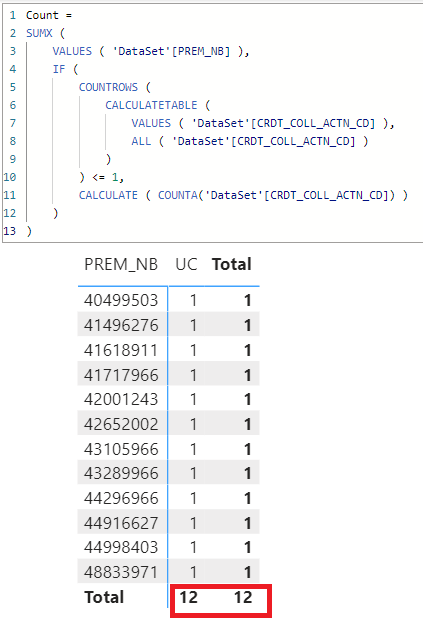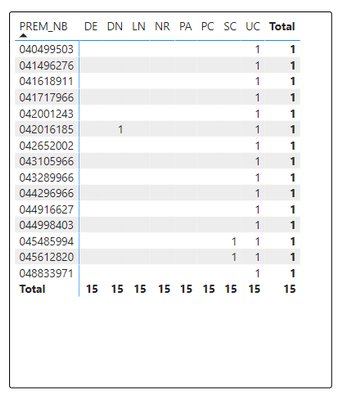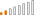cancel
Showing results for
Did you mean:Frequent Visitor

## Need DAX help on Calculate, Count and Filter Functionality

I've tried an IF(AND and CALCULATE(COUNT but I am struggling to find how to exclude the possibility of some variables in a count formula.

I need to return everything in a Column (Action Code) that is "UC" only for a specific prem # (in the left hand column).

In the Matrix. UC is a Count of the Action Code.

In some cases, the Prem # will pull in multiple Action Codes too. So I will get a UC with SC, but I need those omitted and only show UC and null(blank) elsewhere. Maybe I am overthinking the DAX, but I am struggling on this.

I have at least gotten the UC's to all appear in the formula below, but need some assistance in getting the other Action Codes removed. I know I need to Filter out the counts, but unsure how to do this. Such as if UC and SC are both 1 counts, they will be removed from the matrix.

CALCULATE(
COUNTA('DataSet'[CRDT_COLL_ACTN_CD]),
'DataSet'[CRDT_COLL_ACTN_CD] IN { "UC" }
)2 ACCEPTED SOLUTIONSSuper User

@jpaep
Not sure if I correctly understand your requirement. Hope this is what you need. See Attached sample file.``````Count =
SUMX (
VALUES ( 'DataSet'[PREM_NB] ),
IF (
COUNTROWS (
CALCULATETABLE (
VALUES ( 'DataSet'[CRDT_COLL_ACTN_CD] ),
ALL ( 'DataSet'[CRDT_COLL_ACTN_CD] )
)
) <= 1,
CALCULATE ( COUNTA('DataSet'[CRDT_COLL_ACTN_CD]) )
)
)``````Super User

Count =
SUMX (
SUMMARIZE ( 'DataSet', 'DataSet'[PREM_NB], 'Date'[Week] ),
IF (
COUNTROWS (
CALCULATETABLE (
VALUES ( 'DataSet'[CRDT_COLL_ACTN_CD] ),
ALL ( 'DataSet'[CRDT_COLL_ACTN_CD] )
)
) <= 1,
CALCULATE ( COUNTA ( 'DataSet'[CRDT_COLL_ACTN_CD] ) )
)
)

11 REPLIES 11Super User

Hi @jpaep

``````Count =
CALCULATE(
COUNTA ( 'DataSet'[CRDT_COLL_ACTN_CD] ),
KEEPFILTERS ( 'DataSet'[CRDT_COLL_ACTN_CD] = "UC" )
)``````Frequent Visitor

Thanks for the reply. Tried that one as well, but I need the filter to remove anything that has a count in the UC and SC & UC and DN as well. So this gets me halfway there, but not fully. I'm not sure how to write the code as a combination, even if there are duplicate PREM_NB.Super User

@jpaep
Not sure if I correctly understand your requirement. Hope this is what you need. See Attached sample file.``````Count =
SUMX (
VALUES ( 'DataSet'[PREM_NB] ),
IF (
COUNTROWS (
CALCULATETABLE (
VALUES ( 'DataSet'[CRDT_COLL_ACTN_CD] ),
ALL ( 'DataSet'[CRDT_COLL_ACTN_CD] )
)
) <= 1,
CALCULATE ( COUNTA('DataSet'[CRDT_COLL_ACTN_CD]) )
)
)``````Frequent Visitor

Hi @tamerj1

Just have one quick question I need help on with the formula above. While the formula works as intended, I am having one slight issue with the duplicates being omitted from the sum. For example:

This is correct if I am trying to break this down by a weekly count.When I layer month in, it once again works correctly, taking the 8 out of January in a week that stretches into two monthsBut when I condense it to show the month only, I get a smaller number:By doing the quick math it should be 293, but because of the duplicate PREM_NB, there are 55 that are omitted in the 'sum' by month.

Can you help on this? I would think it's an easy fix in the formula, but I am unsure...Super User

Count =
SUMX (
SUMMARIZE ( 'DataSet', 'DataSet'[PREM_NB], 'Date'[Week] ),
IF (
COUNTROWS (
CALCULATETABLE (
VALUES ( 'DataSet'[CRDT_COLL_ACTN_CD] ),
ALL ( 'DataSet'[CRDT_COLL_ACTN_CD] )
)
) <= 1,
CALCULATE ( COUNTA ( 'DataSet'[CRDT_COLL_ACTN_CD] ) )
)
)Frequent Visitor

This seems to have worked for me. Thank you so much!Community Support

Hi @jpaep ,

I'm not sure I understood your question correctly, you mean in the above figure, the 045485994 and 045612820 rows don't want to be displayed in the matrix?

And could you please give me some sample data to test? Please delete sensitive and private data.

Best Regards,

Community Support Team _Yinliw

If this post helps, then please consider Accept it as the solution to help the other members find it more quickly.Frequent Visitor

PREM_NB ORDR_NB ACTN_CD
040499503 049979553 UC
041496276 044247981 UC
041618911 043573555 UC
041717966 042658832 UC
042001243 042886126 UC
042016185 048866316 DN
042016185 041670231 UC
042652002 041132464 UC
043105966 043785616 UC
043289966 045280766 UC
044296966 043610958 UC
044916627 047529882 UC
044998403 042261670 UC
045485994 041311258 UC
045485994 043584388 SC
045612820 046053949 UC
045612820 049905916 SC
048833971 043678617 UC

Here is a mockup of data that could help. There are three duplicate PREM_NB that have separate order numbers. I would need those to not come through in the matrix in Power BI. Even though they have an ACTN_CD of UC, I would want those omitted. I think by doing the count on the ACTN_CD it's not working, although I cannot figure out my filter or anything.Frequent Visitor

I cannot send data on this, sorry. I can try to explain better. If the chart below was an Excel Pivot Table, I would need to filter out the counts of DN and SC, thus eliminating the UC as well. These are duplicate Prem_NB. Although I need all the data, so I cannot remove duplicates either using Power Query. I just do not want the duplicate PREM_NB to show up, so I would be left with a count of 12 in UC.

So while this formula gets me about halfway there, I need a filter(?) formula to take out the counts of the other action codes (DN/SC) and also the UC if they have one. The duplicate prem_nb are 042016185, 045485994, 045612820.

CALCULATE(
COUNTA('DataSet'[CRDT_COLL_ACTN_CD]),
'DataSet'[CRDT_COLL_ACTN_CD] IN { "UC" }
)Frequent Visitor

I've added more context above. If anyone can help it would greatly be appreciated.Continued Contributor

Hi @jpaep ,

It is very to hard to understand. IF possible can you explain the conditions in points.

Regards,

Nikhil Chenna